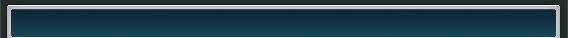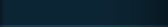Home%    Streaking and Banding Measurement

function [overall_image_mean, overall_image_std, overall_image_snr, column_mean, row_mean, column_std, row_std, column_snr, row_snr, column_streak, row_streak]=image_stats(image);

%   This routine calculates various statistics of an image, including the
%   mean, standard deviation, and signal to noise ratio of the whole image, of
%   columns, and rows.  It also calculates streaking in the column and row
%   directions

[m n]=size(image);
oneD_image=reshape(image,[1,m.* n]);

overall_image_mean=mean(oneD_image);
overall_image_std=std(oneD_image);
overall_image_snr=overall_image_mean./overall_image_std;

%    Calculate mean, standard deviation and SNR of every
%    column and row in the image

column_mean=mean(image);
row_mean=mean(image');
column_std=std(image);
row_std=std(image');
column_snr=column_mean./column_std;
row_snr=row_mean./row_std;

%    Calculate streaking in each column and row
%    Streaks are defined as the difference between a column or row
%    average value and the average of the averages of the two adjacent
%    columns or rows

column_streak=zeros(1,n);
column_streak=abs(column_mean(2:n-1)-(column_mean(1:n-2)+column_mean(3:n))./2);
row_streak=zeros(1,m);
row_streak=abs(row_mean(2:m-1)-(row_mean(1:m-2)+row_mean(3:m))./2);

%   Plot

figure (1)
plot([1:n],column_mean);
figure (2)
plot([1:m],row_mean);
figure (3)
plot([1:n],column_snr);
figure (4)
plot([1:m],row_snr);

 Serious Science LLC            Where science fiction becomes fact.